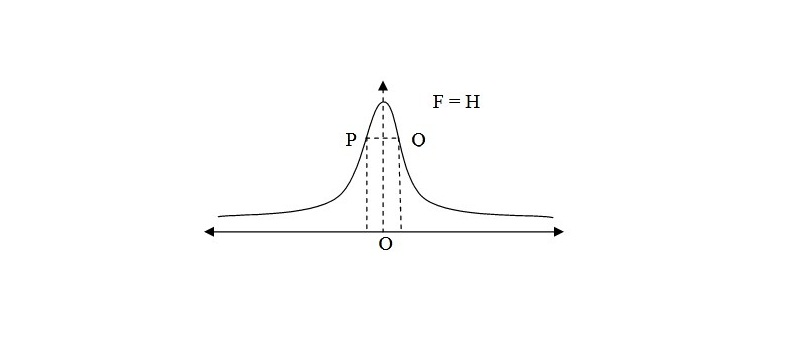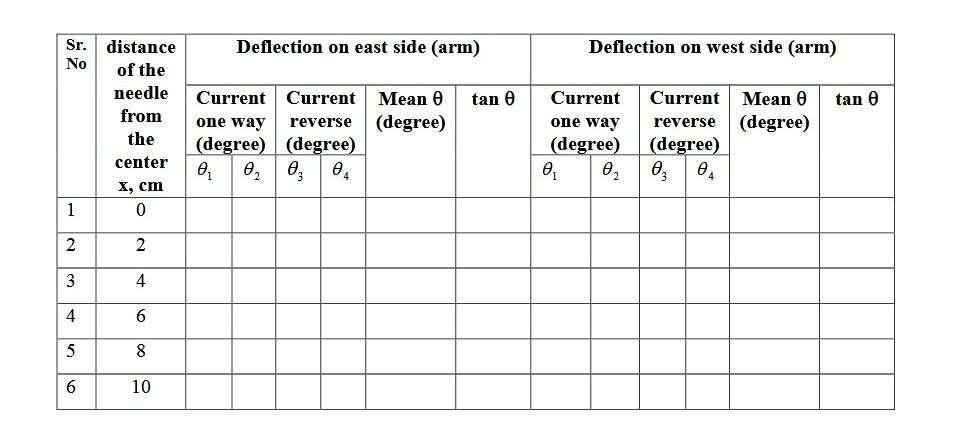# Stewart & Gee Experiment Setup to study the variation of magnetic field

## Objective / Aim of the Experiment

To study the variation of magnetic field and to find the radius of current carrying coil by Stewart & Gee's experiment method.

### Apparatus Required

Tangent Galvanometer of Stewart & Gee'ss type
Battery
Rheostat
A plug key

### Formula Used

(i) Point of inflexoin = (tanθ)m × 0.707

(ii) Radius of coil = Sum of distance between two points on X- axis.

### Theory

This experiment is based on the principle of magnetic effect of current. It consists of circular coil of many turns of thin insulated copper wire. It is fixed with its plane vertical on horizontal bench. A magnetometer compass box is placed inside the coil such that it can slide on the bench in such a way that the center of the needle always lies on the axis of the coil. The distance of the needle from the center of the coil can be read on a graduated scale fixed on the arms of the magnetometer.

### Description of the Apparatus

Stewart & Gee tangent galvanometer consists of a circular coil of thin copper wire with tapings at 5, 25 and 125 (5,50&100) turns. This coil is fixed vertically at the center of one-meter long horizontal bench. A magnetic compass box can slide from the center to either end of the bench along the axis of the coil. The whole length of bench is graduated in millimeter in order to read the distance of the magnetic needle from the center of the coil. The whole set-up is shown in Fig. Next. The magnetic field along the axis of a coil at a point is

F = 2Πn2i / 10(x2 + r2)3/2 ............(1)

Here n is number of turns in the coil, r is the radius of coil, i is the current in ampere flowing in the coil, x is the distance of the point from the center of the coil. If F is made perpendicular to H the earths magnetic field the deflection of the magnetic needle θ is given by H tanθ .......(2)

So, F = 2Πnri2 / 10(x2 + r2)3/2 = H tanθ .......... (3)

### Procedure

1. The magnetometer compass box is placed on the sliding bench so that its magnetic needle is at the center of the coil as shown in Figure in circuit diagram.

2. The coil is set in the magnetic meridian by the whole apparatus in the horizontal plane.

3. The current is adjusted using rheostat Rh so that the deflection of nearly 70o to 75o is produced in the compass needle placed at the center. Both the ends of the pointer are to be read.

4. The direction of the current is reversed and again both the ends of the pointer are read.

5. The mean of four reading in step 3 and 4 given the mean deflection at x.

6. The compass needle is then shifted through 2 cm each time along the axis of the coil and for each position the mean deflection is noted, i, e... steps 3,4 and 5 are repeated for distance x from the center of the coil 2 cm, 4 cm upto 20 cm.

(vii). Perform the above measurement for the west arm in the similar way. This gives deflections θ1, θ2 for the west arm.

(viii). Reverse the current and note the deflections of the pointer θ3, θ4 at the center of the coil. These should be same as θ1, θ2 for x = 0. Repeat steps for the reversed current. This gives θ3, θ4 and for the entire length for the bench.

(ix). The points of inflexion are marked on the curve. The nature of the graph is shown in Figure 2. The distance between the two points gives the radius of the coil.

### Circuit Diagram for Stewart & Gee Experiment Setup### Graph for Stewart & Gee Experiment### Observation Table

Table for measurement of θ### Calculations

A graph is plotted taking distance along x-axis and tanθ along y-axis.
Standard value of the radius of coil = ...........cm.

### Result

(i) The graph between the distance from the center of the coil and tan θ is shown.

(ii) The distance between the points of inflections P & Q is equal to the radius of coil and is of .............. cm.

### Sources of Error and Precautions

1. The coil should be carefully adjusted in the magnetic meridian.
2. All magnetic material should be kept away from the apparatus.
3. The deflection in the coil should be nearly 75o.
4. Both the ends should be read.
5. A smooth curve should be drawn.

### Stewart & Gee's Experiment Viva Questions and Answers

Question-1: What is the magnitude of the field at the centre of the coil?
Answer-1: It is H = 2Πni / 10r, where r is the radius of coilvand n is the number of turns in it. Thus field at the centre increases if we increase no. of turns in the coil.

Question-2: What is the direction of this field?
Answer-2: If in the face of the coil, we look at, the current is flowing in clockwise direction then that face of the coil acts as south pole. Thus direction of the field will be away from us.

Question-3: How does the field vary along the axis of the coil?
Answer-3: It varies as shown in the graph. The points at which curve changes its direction of curvature are called points of inflexion. The distance between them is equal to the radius of coil.

Question-4: Is the field uniform at the centre?
Answer-4: It is uniform only over a very small region at the cntre.

Question-5: How can you get more wide region of uniform field?
Answer-5: By the use of Helmholtz galvanometer which consists of two exactly similar coils placed coaxially.

Question-6: Is it true for an y direction of current in the two coils?
Answer-6: Yes, but current should flow in the two coils in the same direction.

## Related Categories### Spreading Knowledge Across the World

USA - United States of America  Canada  United Kingdom  Australia  New Zealand  South America  Brazil  Portugal  Netherland  South Africa  Ethiopia  Zambia  Singapore  Malaysia  India  China  UAE - Saudi Arabia  Qatar  Oman  Kuwait  Bahrain  Dubai  Israil  England  Scotland  Norway  Ireland  Denmark  France  Spain  Poland  and  many more....